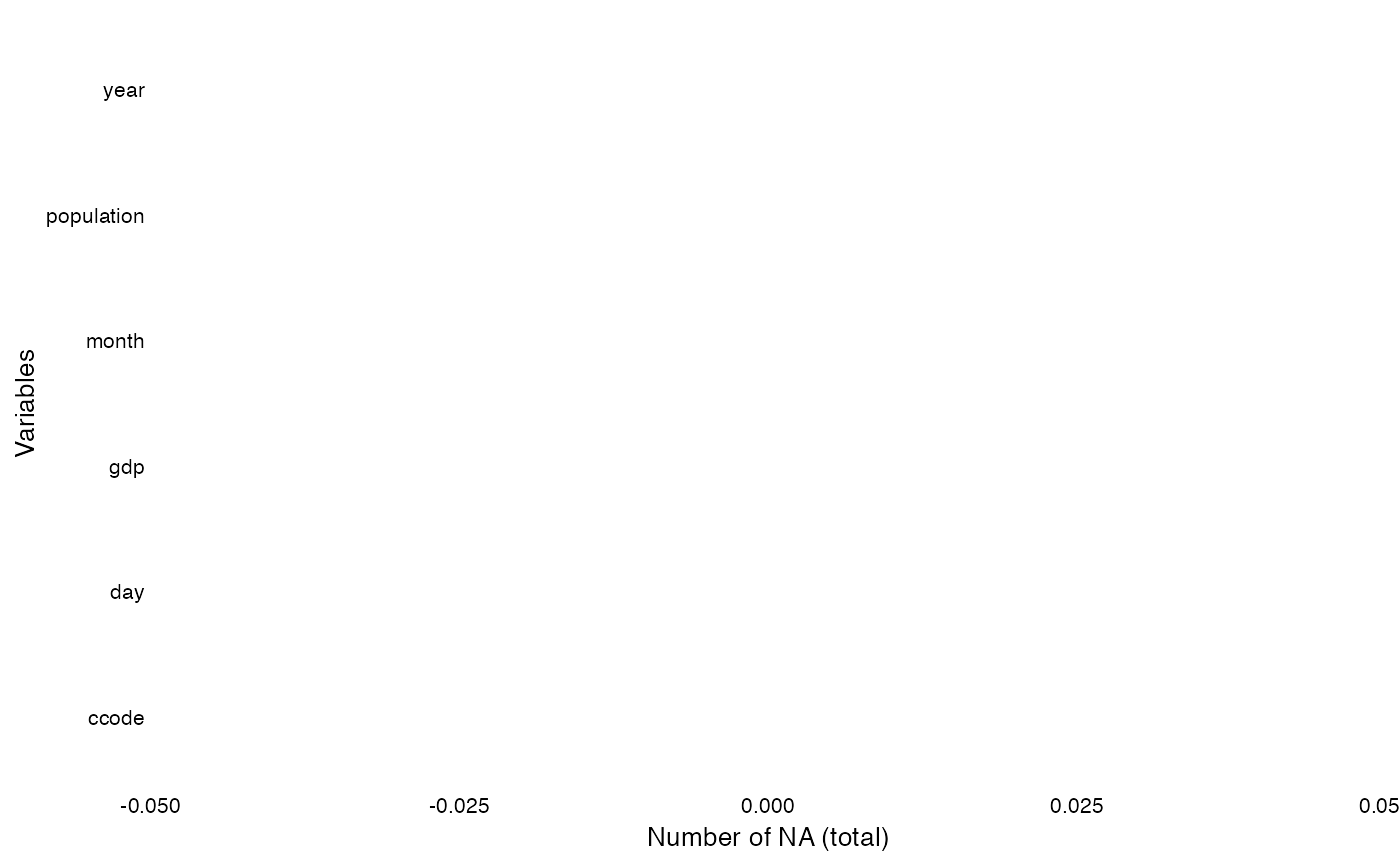This function plots a ggplot to visualize the distribution of NAs across all variables in the data set.

## Usage

overview_na(
dat,
yaxis = "Variables",
perc = TRUE,
row_wise = FALSE,
)

## Arguments

dat

yaxis

Label of your y axis ("Variables" is default)

perc

If TRUE (default) plot returns the number of NAs in percentage

row_wise

If TRUE (FALSE is default) plot return the number of NAs per row

data(toydata)